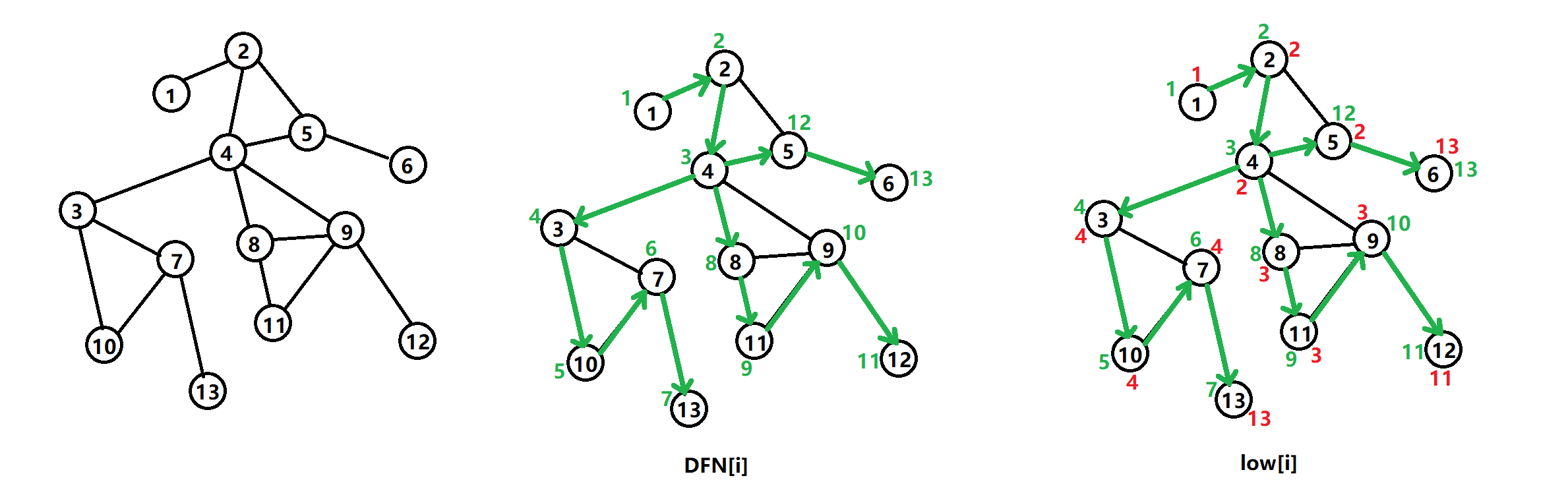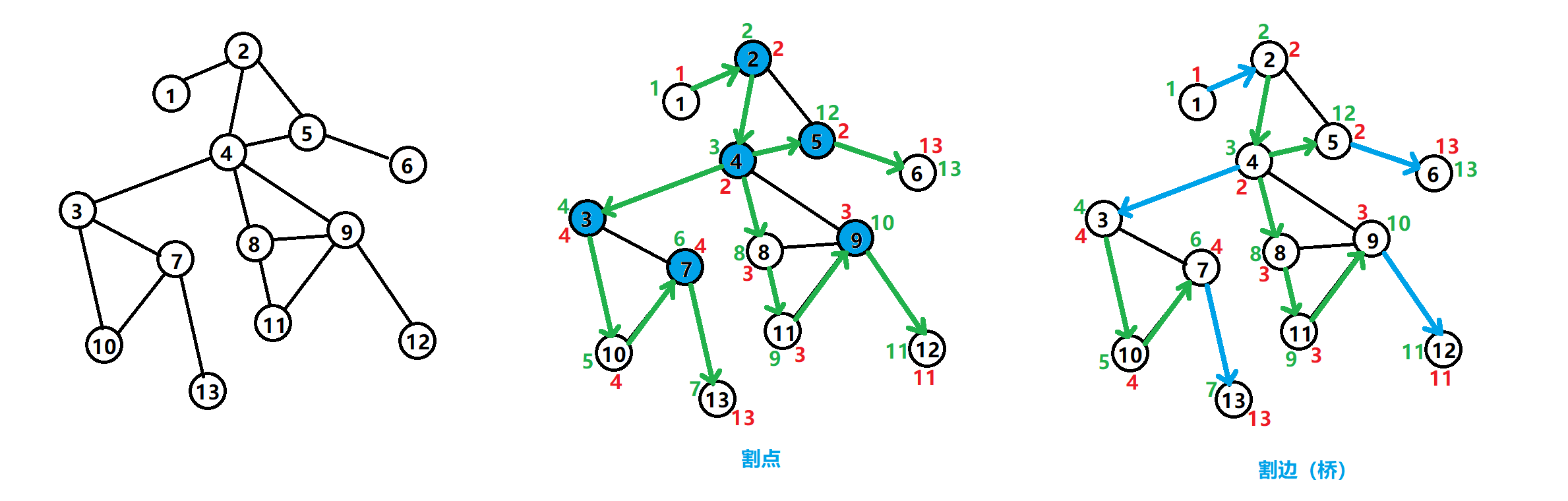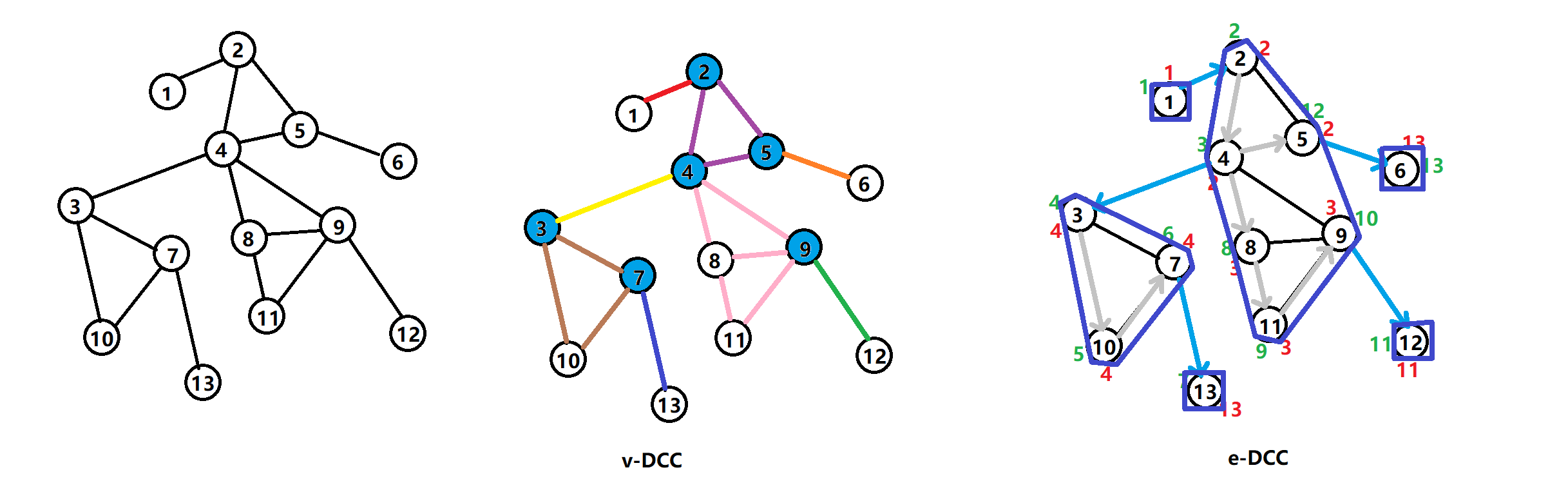Tarjan 算法之无向图

# Tarjan 要素DFS 搜索树：即对图进行 DFS 遍历时所有递归到的边组成的树。

low[x] 定义为以下结点的 DFN 的最小值：（与 Tarjan 有向图中的 low[x] 区分）

1. subtree(x) 中的结点
2. 通过一条不在搜索树上的边能到达 subtree(x) 的结点。

# 无向图的割点与割边## 判定割边 (x,y)

1. 割边一定是 DFS 搜索树上的边.
2. 令 x 是 y 的父结点，则满足 。也就是说，从 subtree(x) 出发，不经过 (x,y) 的前提下无法走到更早访问的结点，则 (x,y) 作为 subtree(x) 与图 G 的唯一连接，即为割边。
``````int dfn[N],low[N],dfn_cnt;
int ans[N],cnt;
void tarjan(int k,int p){//tarjan 找桥
dfn[k]=low[k]=++dfn_cnt;
for(int i=h[k];i;i=e[i].nex){int u=e[i].t;
if(!dfn[u]){tarjan(u,k);
low[k]=min(low[k],low[u]);
if(dfn[k]<low[u])ans[++cnt]=e[i].idx;// 桥
}
else if(u!=p)low[k]=min(low[k],dfn[u]);
}
}
``````

## 判定割点 x

1. 若 x 不为根结点，则要求，存在 x 的子结点 y，满足 . 即 subtree(y) 中的结点最多能走到结点 x，那么将 x 删除就会导致 subtree(y) 与图 G 不连通.
2. 若 x 是根结点，则要求存在至少 2 个的子结点 y，满足 .
``````void tarjan(int u,int p){low[u]=dfn[u]=++dcnt;
int count=0;
for(int i=h[u];i;i=e[i].nex){const int v=e[i].t;
if(!dfn[v]){tarjan(v,u);
low[u]=min(low[u],low[v]);
if(dfn[u]<=low[v])count++;
}
else if(v!=p)low[u]=min(low[u],dfn[v]);
}
if(count-(u==p)>0)ans[++ant]=u;
}
``````

# 无向图的双连通分量## 点双连通分量 ·V-DCC

1. 当结点 x 被第一次访问时，把该结点入栈。
2. 当割点判定条件 成立时，无论 x 是否为根：
1. 从栈顶不断弹出结点，直到 y 被弹出。
2. 刚才弹出的所有结点与结点 x 一起构成一个 v-DCC.

# [POI2008]BLO

## 分析• 割点本身（4 号点）
• 其搜索树上的子结点为根构成的连通块（3,8 为根的搜索树构成的连通块）
• 除上述之外的结点构成一个连通块（1,2,5,6）

## 代码

``````#include<cstdio>
#include<algorithm>
using namespace std;
const int N=100005,M=500005;
int n,m;

struct qxx{int nex,t;};
qxx e[M*2];
int h[N],cnt;
void add_path(int f,int t){e[++cnt]=(qxx){h[f],t},h[f]=cnt;}

int dfncnt;
int dfn[N],low[N],s[N],tp;
int sz[N];// 以 i 为根的搜索树的大小
long long ans[N];
void tarjan(int u,int p){//p 为 u 的父结点
dfn[u]=low[u]=++dfncnt,s[++tp]=u,sz[u]=1;
int count=0,sum=0;// 当前 u 的子结点的子树和
for(int i=h[u];i;i=e[i].nex){const int &v=e[i].t;
if(!dfn[v]){tarjan(v,u);
low[u]=min(low[u],low[v]);
sz[u]+=sz[v];
if(dfn[u]<=low[v]){ans[u]+=(long long)sz[v]*(n-sz[v]);// 统计答案
sum+=sz[v],count++;//count:dfn[u]<=low[v] 的个数
}
}
else low[u]=min(low[u],dfn[v]);
}
if(count-(u==p)>0)ans[u]+=(long long)(n-1-sum)*(1+sum)+n-1;
else ans[u]=2*(n-1);// 不是割点，之前的计算无效
}
int main(){scanf("%d%d",&n,&m);
for(int i=1;i<=m;i++){int a,b;
scanf("%d%d",&a,&b);
}
for(int i=1;i<=n;i++)if(!dfn[i])tarjan(i,i);
for(int i=1;i<=n;i++)printf("%lld\n",ans[i]);
return 0;
}
``````

上一篇Tarjan 之有向图 SCC
tarjan 一直是我认为非常玄学的算法。首先不提它的描述之模糊，dalao 们写的博客之雷同。这算法的应用就很玄学。于是，本蒟蒻今天就来揭开它神秘的面纱…… Tarjan 与 DFS 生成树Tarjan 算法是由 Robert Tarja
2018.12.06最短路算法

2018.12.05
目录